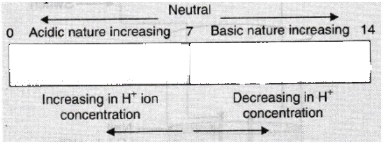# Which is more acidic: a solution of pH = 2 or a solution of pH = 6?

As per the pH scale, it has been divided the pH into 3.
For bases pH >7
For acids pH
For neutral solutions pH = 7
Therefore,  a solution of pH = 2 will be more acidic than a solution of pH = 6.

pH Scale is used to measure acidity or alkalinity of water-soluble substances (pH stands for 'potential of Hydrogen'). It measures H+ concentration in a solution.

• The pH scale ranges from 0 to 14.
• A pH less than 7 is acidic in nature.
• A pH of 7 is neutral.
• A pH greater than 7 is basic in nature.

Given below is the figure showing variations of pH with the change in concentration of H+(aq) and OH-(aq) ions.(Note: The pH of a solution is a measure of the molar concentration of hydrogen ions in the solution and as such is a measure of the acidity or basicity of the solution.

The letters pH stand for "power of hydrogen" and the numerical value for pH is just the negative of the power of 10 of the molar concentration of H+ ions.

To calculate the pH of an aqueous solution you need to know the concentration of the hydronium ion in moles per litre (molarity).

The pH is then calculated using the expression: pH = - log [H3O+]. )

Updated on: 10-Oct-2022

148 Views